## Programming, Hacking, Math, and Art

Recently, I was curious if I could generate a digital radio signal from scratch just using math, and use that signal to control this radio-controlled outlet that I have.

I want to be able to generate modulated digital signals such as this one...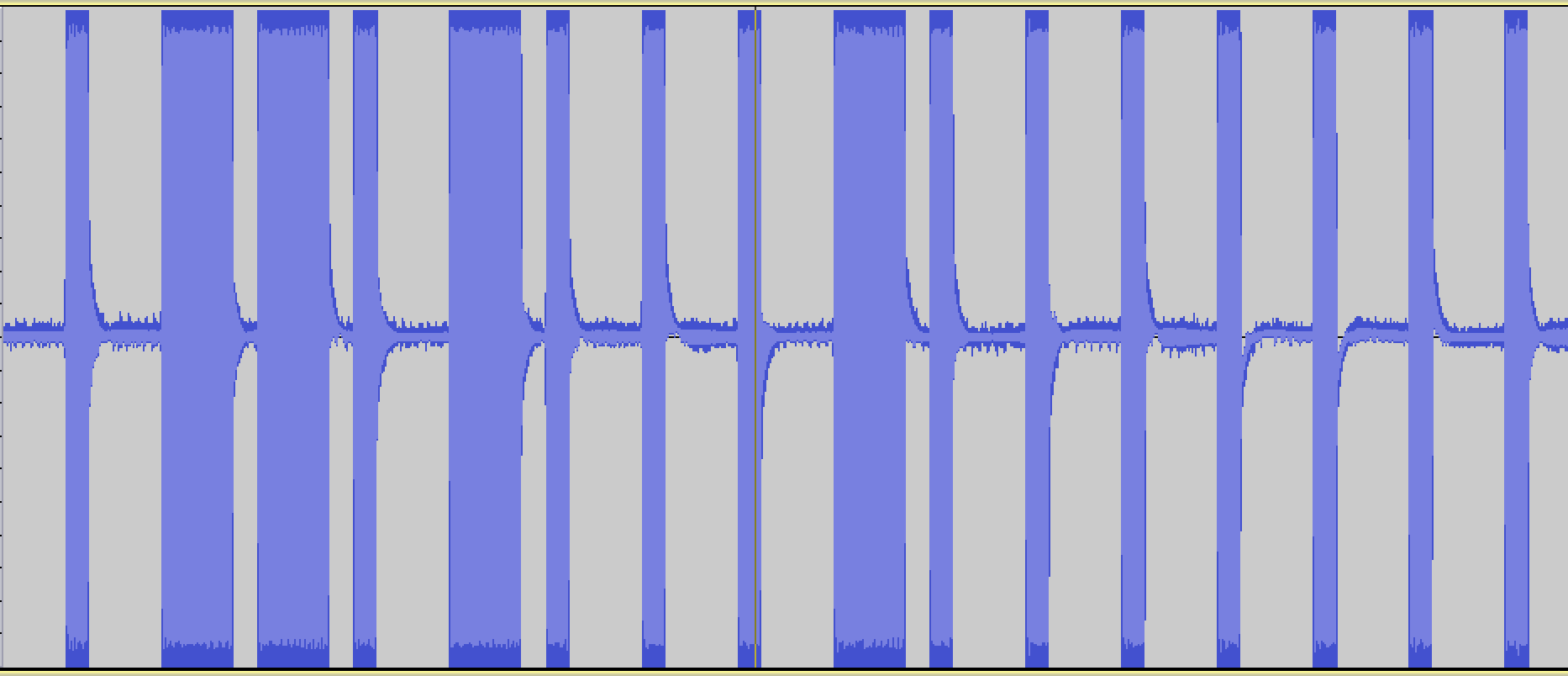This is a binary signal coded with On-off keying (also known as Amplitude-shift keying).

I'm taking the long pulses to mean "1" and the short pulses to mean "0", so this signal is transmitting the digital code, "0110100010000000".

In :
# Imports and boilerplate to make graphs look better
%matplotlib inline
import matplotlib.pyplot as plt
import numpy as np
import scipy
import wave
from ...

# Fourier Transform Notes

The following are my notes from a talk I gave at OSCON 2013. Here is the Github source of these Jupyter/IPython notebooks: https://github.com/calebmadrigal/FourierTalkOSCON

# Sound Analysis with the Fourier Transform and Python

## Intro

1. Welcome
2. Format of talk
• Everything is in iPython Notebook (on Github)
• You don't need to take notes
• Please save questions for the end
3. Why this is interesting
• Sound processing is big - natural human-machine interfaces (e.g. Siri)
• Noise reduction, Compression, feature extraction (e.g. speech)
• Understanding our universe better (Superposition, Harmonics, Sound timbre)

## Overview

• The Nature of Waves
• The Fourier Transform
• Fast Fourier Transform (FFT) in Python
• Audio analysis

In :
%matplotlib inline
import matplotlib.pyplot as plt
import numpy as np
import ...

# Calling synchronous code from asyncio

I recently needed to call some synchronous code from asyncio. Thankfully, asyncio provides the run_in_executor function, which runs the specified function in a different thread. Here is an example of using it:

# Algorithms in Python

I recently decided to brush up on my algorithms and data structures by writing them in Python. Though these are not optimized, they could be helpful for reference. Here is the Github repo: https://github.com/calebmadrigal/algorithms-in-python.

A some of the algorithms included:

# Recursion with asyncio

Recursion is awesome, but has the downside of growing the stack, which can limit its usefulness. Some languages like Scheme, however, have Tail-call optimization, which lets programmers write Tail-recursive functions that don't grow the call stack. Python does not have Tail-call optimization (TCO), but with asyncio, we can have something like Tail-call optimization. Basically, this method uses the asyncio event loop like a trampoline function.

Example:

# Fuel efficiency difference cost

I recently was car shopping, and I had the question about gas mileage: "How much does, say, the difference between 25 mpg and 30 mpg cost?"

To answer this question, I did a bit of analysis using IPython Notebook...

# Goal

• To find how much different fuel efficiencies cost in terms of dollars per year.
• I want to estimate this value for the next 10 or so years.

# Assumptions

• I'm estimating the average price per gallon of gas (over the next 10 years) to be $4.50. It is around$3.60 now, I adjusted for inflation, and counter-adjusted for the time-value of money.
• I'm estimating that we will drive about 14,000 miles per year (based on the last 3 years).

# Data

In :
price_per_gallon = 4 ...

# Display List as Table in IPython Notebook

IPython Notebook provides hook methods like _repr_html_ which can be defined by Python objects to allow richer representations. Here's an example:

In :
class ListTable(list):
""" Overridden list class which takes a 2-dimensional list of
the form [[1,2,3],[4,5,6]], and renders an HTML Table in
IPython Notebook. """

def _repr_html_(self):
html = ["<table>"]
for row in self:
html.append("<tr>")

for col in row:
html.append("<td>{0}</td>".format(col))

html.append("</tr>")
html.append("</table>")
return ''.join(html)

In :
import random
table = ListTable()
table.append(['x', 'y', 'x-y', '(x-y)^2'])
for i in xrange(7):
x = random.uniform(0, 10)
y = random.uniform(0, 10)
table.append([x, y, x-y, (x-y)**2])
table

Out:
 x y x-y (x-y ...

# How To Draw Lines With Matplotlib

Simple example to show how to draw lines with Matplotlib (in IPython Notebook).

ipython notebook --pylab inline


In :
import matplotlib.lines as lines
fig, ax = plt.subplots()

fig.set_size_inches(6,6)          # Make graph square
scatter([-0.1],[-0.1],s=0.01)     # Move graph window a little left and down

line1 = [(0,0), (1,0)]
line2 = [(0,0), (0,1)]

# Note that the Line2D takes a list of x values and a list of y values,
# not 2 points as one might expect.  So we have to convert our points
# an x-list and a y-list.
(line1_xs, line1_ys) = zip(*line1)
(line2_xs, line2_ys) = zip(*line2)

plot()
show()

[Image_Link]data:image ...

# Opposite of zip function in Python

Is there a way to do the opposite of what the zip() function does? It turns out, there is - the zip() function with list unpacking...

### Normal zip() usage

In :
x = [1,2,3,4,5]
y = [10,20,30,40,50]
points = zip(x,y)
points

Out:
[(1, 10), (2, 20), (3, 30), (4, 40), (5, 50)]


### Reverse of normal zip() with list unpacking

By using list unpacking (by using the asterisk before the list), zip can effectively act in reverse...

In :
zip(*points)

Out:
[(1, 2, 3, 4, 5), (10, 20, 30, 40, 50)]


### Example of usefulness

In :
import random
rand = lambda: random.gauss(mu=1, sigma=1)
points = [(rand(), rand()) for i in xrange(1000)]

# Make the graph square
fig = plt ...

# Monte Carlo In Python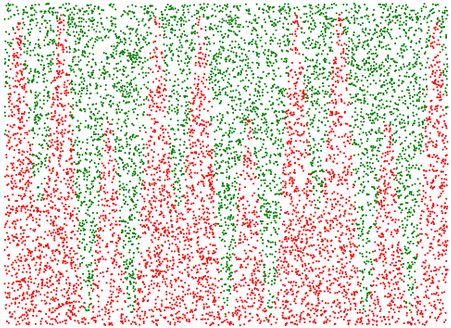# Monte Carlo Estimation

Monte Carlo Estimation is a method of numerically estimating things which we don't (or can't) calculate numerically by randomly generating samples. In this IPython Notebook, I'm going to use Monte Carlo Estimation to estimate:

## Monte Carlo Estimation of Area

Let's use Monte Carlo Estimation to estimate the area onder this curve (from 0 to 10):

$y = 5 * \sin(6~x) + \sin(2~x) + 7$

### Here are the basic steps:

1. Define a rectangle which encloses the part of the curve for which we want to find area.
2. Randomly generate points within that region.
3. Find which of the randomly generated points are under the curve by checking them against the equation of the function ...

# Wordpress to Pelican Reasons

I recently migrated my blog from Wordpress to Pelican. Pelican is a static-site generating blog system which is written in Python and uses Jinja2 for templating. I'll probably do a post about the migration process later, but for now, I'll just give my reasons for moving to Pelican...

### Data Longevity

I didn't want my blog data stored in a database; I vastly prefer it being stored in version-controlled Markdown format.

### Markdown

• I wanted to write blog posts in Markdown (which is possible in Wordpress, but Wordpress isn't designed to use Markdown).
• I also get to use vim to write my blog posts now, which is much nicer than the Wordpress editor.

### Language

• I hate PHP, but love Python. It worried me that my blog ...

# IPython Notebook on a VPS

## Overview

This is a guide to set up IPython Notebook on a Server - specifically, on a DigitalOcean VPS. This will allow you to access your iPython Notebooks from anywhere.

### Overview of Steps:

• Set up a domain name
• Get a VPS
• Install IPython Notebook (and all dependencies)
• Configure IPython Notebook to run in a server mode
• Make IPython Notebook start automatically

## Create a domain

Go to http://freedns.afraid.org and click "Setup an account here" Go through the signup form Click on the activation link they send to your email This will bring you back to their site; Click the link you see there called "Add a subdomain" Here is how I filled out the form:### Notes:

• Leave the Destination alone for now, and leave the ...

# Big graphs in IPython Notebook

I've been doing a good bit of graphing in IPython Notebook recently, and I often wanted to make the graphs larger. I also often wanted to label the graph axes. So I wrote this simple function and have been using it a lot.

# Graphing helper function
def setup_graph(title='', x_label='', y_label='', fig_size=None):
fig = plt.figure()
if fig_size != None:
fig.set_size_inches(fig_size, fig_size)
ax.set_title(title)
ax.set_xlabel(x_label)
ax.set_ylabel(y_label)


Here's a demo of using it...

In :
x = linspace(0, 2 * pi, 1000)
y = 5 * sin(1 * 2*pi*x) + 4 * sin(2 * 2*pi*x)


## Without setup_graph()

In :
_ = plot(x, y)

[Image_Link]data:image/png;base64,iVBORw0KGgoAAAANSUhEUgAAAXAAAAD9CAYAAAClQCyNAAAABHNCSVQICAgIfAhkiAAAAAlwSFlz AAALEgAACxIB0t1+/AAAIABJREFUeJztnXl0lfWdxp+sJGwhCSEBguxLAgiRbaZgDY7UbWiVoiO4 DVin59TpKY5HW3umLWhxGdsZRTye0aN10E5xWnuKWkWgNEIRZAmLIrImEFYhkEA2bpJ754+vL7m5 ee ...

# Pandas Into

I've recently been playing with the pandas Python data analysis framework while reading the Python for Data Analysis book (which is written by the author of the pandas library). Here are 2 of my IPython Notebooks that demonstrate some of the basics:

And here's a little screenshot of what it looks like in IPython Notebook: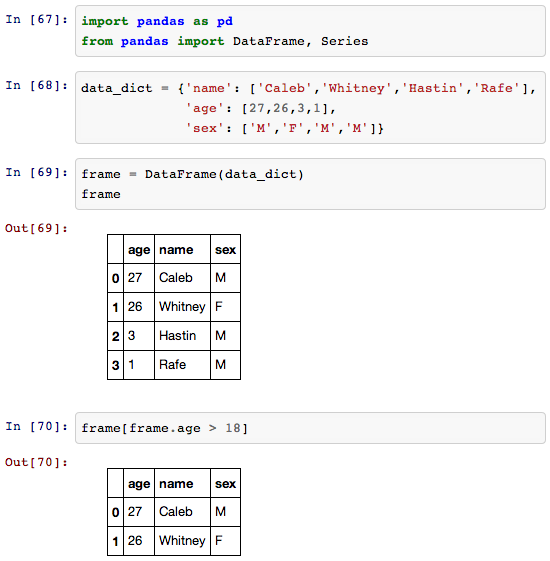# Finding the Golden Ratio

I recently was exploring the Golden Ratio. I was really surprised what a simple (and recursive) relationship it comes from: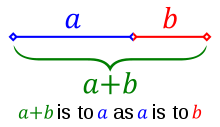Starting with this this image, here is the derivation of the golden ratio...

From this relationship we see in this image, we can derive this equation: $\frac{a}{b} = \frac{a+b}{a}$

And if we then let b=1 (so we can find the ratio of a to 1), we get: $a = \frac{a+1}{a}$

Then, with this formula, we can define a function: $f(a) = \frac{a+1}{a}$

If we then find the fixed-point in this function (so that f(x) = x), then we will have found a solution to $a = \frac{a+1}{a}$

A simple way to think about the fixed-point of ...

# How to graph with IPython Notebook

IPython Notebook / Matplotlib / Pylab / Numpy is great for graphing (among other things). Below is a simple demo of how to graph with it.

To run IPython Notebook, use this command: ipython notebook --pylab inline

Here's a screenshot: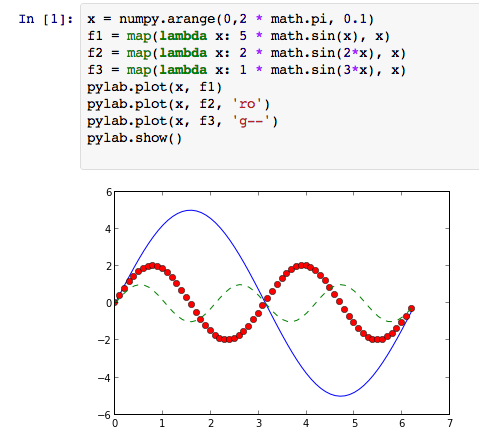Here's an embedded IPython Notebook showing a slightly easier way:

In :
x = linspace(0, 2*pi, 42)
f1 = 5 * sin(x)
f2 = 2 * sin(2*x)
f3 = 1 * sin(3*x)
plot(x, f1)
plot(x, f2, 'ro')
plot(x, f3, 'g--')
show()

[Image_Link]data:image/png;base64,iVBORw0KGgoAAAANSUhEUgAAAXAAAAD9CAYAAAClQCyNAAAABHNCSVQICAgIfAhkiAAAAAlwSFlz AAALEgAACxIB0t1+/AAAIABJREFUeJzt3Xl8TNf7B/BPQlKNlIomoULFiErEkuKrtAhKELtUJZRa f61+EVW1BUFjXxqprahqqaXWCIlQEkuoJWopEiWIvYglmyxzfn+cLw2SySz3zrl35nm/Xnk1mdy5 94nOPHPuOc85x4YxxkAIIUR1bEUHQAghxDiUwAkhRKUogRNCiEpRAieEEJWiBE4IISpFCZwQQlTK 5AT+8OFDBAQEwNPTE15eXjhy5IgUcRFCCClGSVNPMHz4cLRv3x4bN25EXl4eMjIypIiLEEJIMWxM mcjz6NEj+Pj44PLly1LGRAghRA8mtcBTUlLg7OyMfv364dSpU6hfvz7Cw8Ph4OAAALCxsZEkSEII sTb6tK1N6gPPy8tDYmIihgwZgsTERJQuXRozZsx4JQi1fk2aNEl4DBS/+DisMX41x24J8evLpATu 5uYGNzc3NGzYEAAQEBCAxMREU05JCCFETyYl8AoVKqBy5cpITk4GAOzZswe1atWSJDBCCCG6mVyF EhERgV69eiEnJwcajQYrV66UIi5F8PX1FR2CSSh+sdQcv5pjB9Qfv75MqkIp9uQ2Ngb15xBCCNE/ d9JMTEIIUSlK4IQQolKUwAkhRKUogRNCiEpRAieEEJWiBE4IISpFCZwQQlSKEjghhKgUJXBCCFEp SuCEEKJSlMAJIUSlKIETQohKmbwaITFNZiZw7hzg4ACULw84OQF2dqKjIoSoASVwM2IMSEkBDh/+ 9+vCBaB6deDpU+D+fSAtDXB05Mn82deHHwKffgpUqSL6LyCEKAklcJkxBmzbBqxaxRO2rS3QuDH/ CgwE6tcHSpX693itFnj0iCfz+/eBO3eA6GjAxweoWxfo2xfo3p0neUKIdaP1wGXCGBATA0ycCOTm AiNHAs2a8Va0MXs9Z2cDUVH8g+DAAaBzZ6BPH6BFC/6hQAixHPrmTkrgMti3DwgJ4d0hU6YA3bpJ m2Tv3AHWruXJPCcH+PFHoFEj6c5PCBGLErgACQnAhAnA1atAaCjvIilRQr7rMQb89hswbBjQuzf/ sHBwkO96hBDzoB15zOjxY+CTT3jCDgoCzp/nCVXO5A3wrpgePYAzZ4CbN3kf+f798l6TEKIclMBN dOEC774oVw5ISgIGDDB/GaCzM/Drr8CcOfxD5L//BdLTzRsDIcT8KIGbYOtWoGlTPkC5ZMmL1SQi ...

# Pyknon Intro, Chords, and Intervals

I've continued on my (hopefully) short-term fascination with music+math (it's fun, but really bad for productivity). So I found this great library for generating music in Python called Pyknon. And it can be installed using pip: pip install pyknon.

I wrote a little python script just to help me understand some concepts in music theory like intervals and chords. It is meant to be read top to bottom (which is why I intersperse functions and variables throughout). It is NOT written in good modular form (in general, I don't recommend writing python like this). This code can also be used as an intro to the pyknon library.

Behold:

from pyknon.genmidi import Midi
from pyknon.music import NoteSeq, Note, Rest

####### First we'll generate ...

# Music Theory and Math Notes

For the last day, I've done some reading on music theory (trying to understand why things are the way they are in terms of math). Here are my raw unedited notes:

## Important musical ratios:

• Unison: 1:1 frequency
• Octave: 2:1 frequency
• 12 semitone increase
• Fifth: 3:2 frequency (i.e. multiply by 1.5)
• 7 semitone increase
• Semitone: 2^(1./12) (12th root of 2) - about 1.059 - this is the "half-step" distance, so you can multiply the frequency of F by 1.059 to get the frequency for F#

## Scales and keys

• Chromatic scale: 12 notes (list of all semitones in an octave)
• Diatomic scale: 7 notes
• To be in a "key" (like C-major) means to select 7 notes from the 12 notes in the ...

# Reduce with Python and Clojure

I was just playing around writing an annuity calculator function. Here is my first version:

def calculate_annuity(years, interest=0, addition_per_year=0, starting_amount=0):
result = []
running_total = starting_amount
for year in range(years+1):
result.append(running_total)
running_total = (running_total + addition_per_year) * (1 + interest)
return result


Here is a 2nd version, written using reduce instead of a loop:

def calculate_annuity2(years, interest=0, addition_per_year=0, starting_amount=0):
return reduce(lambda result,addition: result + [(result[-1] + addition) * (1 + interest)],


And here is a version in Clojure (basically a direct translation of the Python one:

(defn annuity [years interest addition_per_year init]
(reduce
#(conj %1 (* (+ (last %1) %2) (+ 1 interest)))
[starting_amount]


Here are some example use cases:

; Find investment returns by year for a \$1000 investment ...

# Facial Detection with OpenCV and Python

I was able to get some basic facial detection working in OpenCV with Python. Here's what it looks like: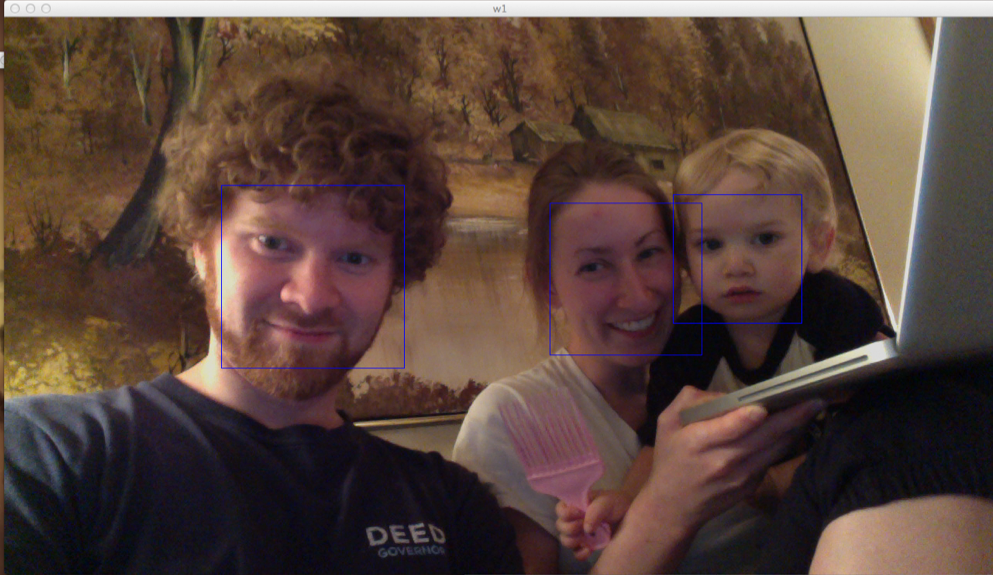And here is the 34-line python script to draw boxes around all detected faces in live video:

import cv

CAMERA_INDEX = 0

def detect_faces(image):
faces = []
detected = cv.HaarDetectObjects(image, cascade, storage, 1.2, 2, cv.CV_HAAR_DO_CANNY_PRUNING, (100,100))
if detected:
for (x,y,w,h),n in detected:
faces.append((x,y,w,h))
return faces

if __name__ == "__main__":
cv.NamedWindow("Video", cv.CV_WINDOW_AUTOSIZE)

capture = cv.CaptureFromCAM(CAMERA_INDEX)
storage = cv.CreateMemStorage()
faces = []

i = 0
while True:
image = cv.QueryFrame(capture)

# Only run the Detection algorithm every 5 frames to improve performance
if i%5==0:
faces ...

# Intro to OpenCV with Python

I have started my journey into the world of OpenCV using Python on my Mac. These are my first steps with it.

# Installing OpenCV for Python on the Mac

# Install numpy as a prerequisite
sudo port install numpy

# Install OpenCV with the Python API
sudo port install opencv +python27


(The port command is part of Macports)

# Capturing an images from a webcam

  1 2 3 4 5 6 7 8 9 10 #!/usr/bin/env python2.7 import cv cv.NamedWindow("w1", cv.CV_WINDOW_AUTOSIZE) camera_index = 0 capture = cv.CaptureFromCAM(camera_index) frame = cv.QueryFrame(capture) cv.SaveImage("pic.jpg", frame) 

(I tested this with the iSight camera on my Macbook Pro)

# Displaying live video

  1 2 3 4 5 6 7 8 9 10 11 #!/usr/bin/env python2 ...

# First look at Pylab/Matplotlib

Since I've been getting into Machine Learning/Artificial Intelligence recently, I've been looking at various computing environments recently. Some of the contenders are:

• MATLAB - The traditional software stack for doing machine learning and statistical analysis
• GNU Octave - An open-source MATLAB clone.
• R - An open source clone of a statistical computing environment called S.
• Julia - A language for doing statistical analysis. The goals are to compete with Matlab and R.
• Matplotlib/Pylab/SciPy/NumPy - see below

Of these, I've tried Octave and Matplotlib. Matplotlib/Pylab is the software stack consisting of:

• IPython - an interactive REPL for Python with things like tab completion
• Matplotlib - a graphical plotting library
• NumPy - a matrix library
• SciPy - a collection of scientific and mathematical algorithms

I've only played with Matplotlib/Pylab ...

# Probability and Statistics book for Python programmers

This seems like a nice, concise book on probability and statistics for Python programmers: Think Stats.

# Linear Regression with Gradient Descent

I'm taking the Stanford Machine Learning class. The first algorithm we covered is Linear Regression using Gradient Descent. I implemented this algorithm is Python. Here's what it looks like:

import subprocess

def create_hypothesis(slope, y0):
return lambda x: slope*x + y0

def linear_regression(data_points, learning_rate=0.01, variance=0.001):
""" Takes a set of data points in the form: [(1,1), (2,2), ...] and outputs (slope, y0). """

slope_guess = 1.
y0_guess = 1.

last_slope = 1000.
last_y0 = 1000.
num_points = len(data_points)

while (abs(slope_guess-last_slope) > variance or abs(y0_guess - last_y0) > variance):
last_slope = slope_guess
last_y0 = y0_guess

hypothesis = create_hypothesis(slope_guess, y0_guess)

y0_guess = y0_guess - learning_rate * (1./num_points) * sum([hypothesis(point) - point for point in data_points])
slope_guess = slope_guess - learning_rate * (1./num_points) * sum([ (hypothesis(point) - point) * point for point ...

# Tail call optimization in Python

Since I've been getting into functional programming more recently, the fact that Python doesn't do tail-call optimization has been bothering me. So I did a bit of searching, and found this gem: Tail Call Optimization Decorator.

Here is a snippet from it:

import sys

class TailRecurseException:
def __init__(self, args, kwargs):
self.args = args
self.kwargs = kwargs

def tail_call_optimized(g):
"""
This function decorates a function with tail call
optimization. It does this by throwing an exception
if it is it's own grandparent, and catching such
exceptions to fake the tail call optimization.

This function fails if the decorated
function recurses in a non-tail context.
"""
def func(*args, **kwargs):
f = sys._getframe()
if f.f_back and f.f_back.f_back \
and f.f_back.f_back.f_code == f.f_code ...

# Standard Deviation in Python

I just wanted to go through the process of calculating standard deviation today, and this is how I did it in Python. Python makes such a nice calculator :)

>>> s = [2,4,4,4,5,5,7,9]
>>> def average(s): return sum(s) * 1.0 / len(s)
...
>>> avg = average(s)
>>> avg
5.0
>>> variance = map(lambda x: (x - avg)**2, s)
>>> variance
[9.0, 1.0, 1.0, 1.0, 0.0, 0.0, 4.0, 16.0]
>>> average(variance)
4.0
>>> import math
>>> standard_deviation = math.sqrt(average(variance))
>>> standard_deviation
2.0
>>>


# Django on Hostmonster

I just finished going through the process of installing and configuring Django (with FastCGI) on Hostmonster's Shared hosting. It was more painful than I expected, so I decided write a post about how I got it working...

First, I wanted to create a subdomain which would host my django stuff. In order to do this, I created a subdomain using the cPanel (the item is called "Subdomains"). This created the directory and a basic .htaccess file (along with a few error pages).

I then went through the basic processes described on these pages:

When I finished these guides, I kept getting a 500 error. I eventually found that I can access the error log ...

# Consuming web service in Python with SUDS

import datetime
from suds.client import Client
url = "http://localhost:9080/dataplanws/DataPlanWebService/WEB-INF/wsdl/DataPlanWebService.wsdl"
client = Client(url)
print client.service.hello("Caleb")